# Python Functions

## Introduction

In this chapter, we will learn to use Python Functions.

## Function

A function in programming terms can be defined as “a block of the reusable, managed code block that is used to perform any certain single operation.

Functions provide better functioning for your application and a high degree of code reusability.

We can also call it a machine that gives desired outputs depending on our input. Something like the following: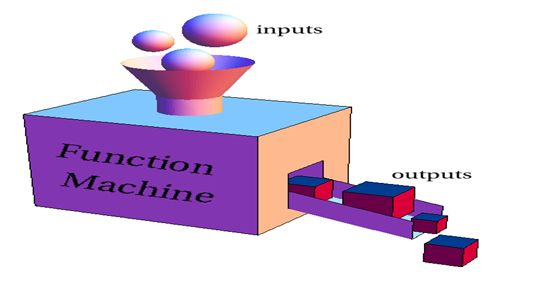Python contains several types of built-in functions such as print(), del, len, and so on. These types of functions are called built-in functions, but still, like several other programming languages, they provide complete freedom to create your own functions based on your requirements. These types of functions are called user-defined functions.

### Creating a Function

As I said earlier, you can create your own built-in functions. So let's have a look at how to create a function.

The following are the set of rules that you need to follow when creating a function:
• A function block in Python begins with a keyword def.
• A function name after that keyword "def" and parentheses "()".
• Any input parameter or arguments for a specific functionality should be placed between these parentheses.
• An optional statement, "fun_docstring" is followed by the parentheses, but it's optional.
• For accessing and invoking a function In Python, we should use a colon ":".
• Then your function or some set of code for performing a certain operation.
• Finally a return statement.

### Structure

Let's have a look at the structure:

def funcname (parameters):
# “func_docstring”
[func_suite]
return [expression]

Example

Creating several basic functions.
2.       print ("Adding : %d + %d" % (a, b))
3.       return a + b
# Function: Subtract
1. def subtract(a, b):
2.      print ("Subtracting : %d - %d" % (a, b))
3.      return a - b
# Function: Multiply
1. def multiply(a, b):
2.       print ("Multiplying : %d * %d" % (a, b))
3.       return a * b
# Function: Divide
1. def divide(a, b):
2.      print ("Dividing : %d / %d" % (a, b))
3.      return a / b
4.      print ("Let's use the functions created by us")
# Calling Functions
2. B = subtract(56)
3. C = multiply(65)
4. D = divide(55)
5.
6. print ("A : %d | B : %d | C : %d | D : %d" % (A, B, C, D))
Output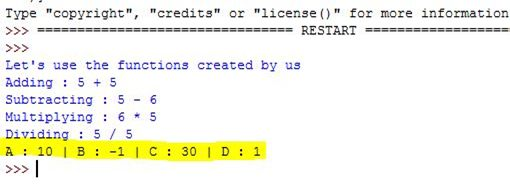### Calling a Function

Creating a function is just 50% of the entire work, moreover, for performing certain operations you need to call that function later in your code snippet. So let's have a look at how it can be done in Python.

Once the basic block of function is formed, here are the approaches that you need to use when calling a function in Python.

## Function Calling | Approaches

Here are some approaches: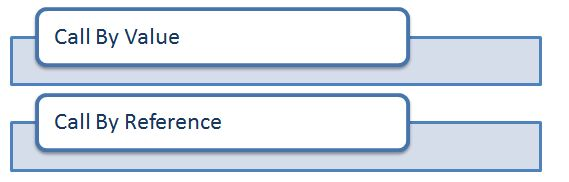• Call By value

- The most common strategy of parameter or argument passing is call-by-value, sometimes it is also called pass-by-value. This strategy is used in several programming languages like C, C#, Java, C++, and so on.

- In the call by value approach, the argument expression is evaluated first and the result of this evaluation is bound to the corresponding variable in the function. So, if the expression is a variable, a local replica of its value will be used.

- Here's how it works: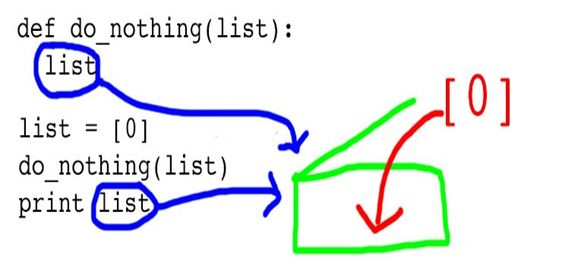Explanation

Here a replica of the list (all the values and elements in it) is used for functionality via passing a value.

• Call By Reference

- In a call by reference approach, a function gets an implicit reference to the argument rather than a copy of its value. As a result, the function can modify the argument and the value of the variable in the caller's scope can be changed.

- This strategy is also known as pass by reference and is used in several programming languages, such as C, C#, Java, C++, and so on.

- The advantage of the call by reference is greater time and space-efficient because arguments do not need to be copied.

- Here's a sample functionality: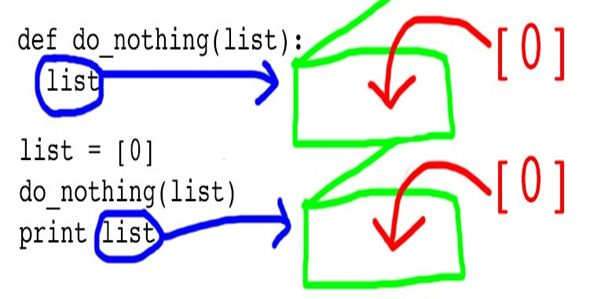Explanation

In this approach, it works slightly differently. Here there are two locations and for both of the locations, only a reference is being used instead of the replica itself.

## Function Calling | Arguments

In Python you can call a function using these types of arguments: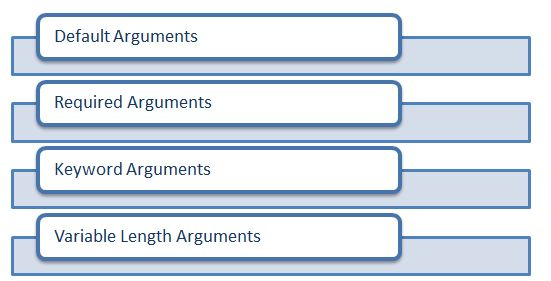• Default Arguments

As the name suggests, a default argument always assumes a default value in case there is no value provided in the function call for a specific argument.

Example
1. def empinfo(name = "ABC", designation):
2.     print ("Name : ", name)
3.     print ("Designation : ", designation)
4.     return;
5.
6. empinfo( designation = "Dev" )
OutputNow let us see some changes in the code and call the function again for a different pair or a different value:
1. def empinfo(name = "XYZ", designation):
2.      print ("Name : ", name)
3.      print ("Designation : ", designation)
4.      return;
5.
6. empinfo( name = "ABC", designation = "Dev" )
7.
8. empinfo( designation = "Dev" )
Output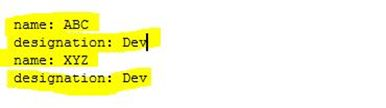• Required Arguments

Required arguments are those that are passed to a function in a proper positional/hierarchical order. In Python, the number of arguments in the function call should be exactly the same as the function definition.

Example
1. def callme( str ):
2.      # "This prints a passed string into this function"
3.      print (str)
4.      return;
5.
6. callme()
Output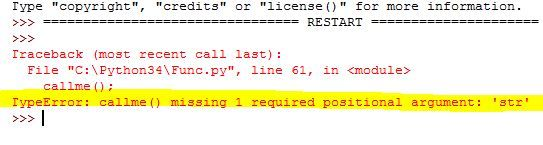Explanation

As I explained in the preceding paragraph, a required argument needs to pass one argument in the callme function and we didn't do that. That's why the snippet is showing an error.

But if you pass an argument then it will produce an output for sure.

• Keyword Arguments

Keyword arguments are somehow similar to the function calls. Whenever you use keyword arguments in a function call, it is identified by the parameter name directly.

There are many advantages to the use of this approach, these are:
- it allows you to skip the arguments.
- Even you can place the arguments out of order.
- You can also make keyword calls (using function name).

Example
1. def callme( str ):
2.      print (str)
3.      return;
4.
5. callme( str = "Calling that function");
Output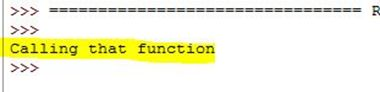Explanation

If you haven't yet gotten the theme then don't worry. Let's explore it with a similar type of example as we used for the default try arguments, to provide you a clear view.
1. def empinfo(name , designation):
2.      print ("Name : ", name)
3.      print ("Designation : ", designation)
4.      return;
5.
6. empinfo( name = "ABC", designation = "Dev" )
Output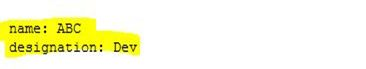(I hope now it will be clear to you guys.)

• Variable Length Arguments

All the three above specified arguments are enough to perform any operation, but still, you may need to process a function for more arguments than you specified. For that, here comes the concept of Variable Length Arguments.

Example
1. def callme( arg, *vartuple ):
2.      print ("India??")
3.      print (arg)
4.      for var in vartuple:
5.           print (var)
6.      return;
7.
8. callme( "Democracy""or""Gerontocracy")
Output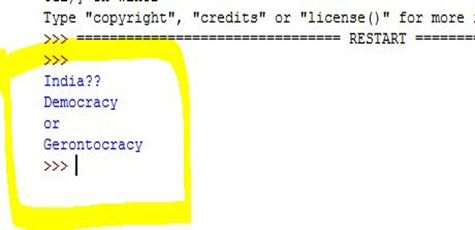## Conclusion

In the next chapter, we will learn to use Python List.
Next » Python List